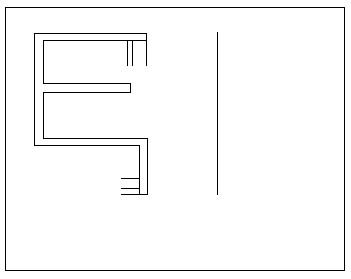## SSC CGL Reasoning Test 7

Instructions

For the following questions answer them individually

Question 1

Five children are standing in a row. O is third from M who is standing left of N, and P is on the left of Q who is second from N. What is the position of P from right?

Question 2

Which answer figure is the exact mirror image of the given question figure when the mirror is held from the right at PQ ?Question 3

For the following questions
Find the odd word/letters/number pair from the given alternatives

Question 4

In the following question, select the odd word from the given alternatives.

Question 5

Identify the diagram that best represents the relationship among the given classes.

Animal, Leopard, Lion